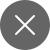# Explaining Lasso and Ridge Regression

4+
##### One of the major problems that we face in machine learning is Overfitting. This problem tends to reduce the performance of our model. Overfitting occurs when our machine learning model learns noise with useful information. Due to which model gives good results on training data but poor results on unseen data. In this article, we are going to discuss some regularization techniques that help in avoiding overfitting.

Let me first tell you what regularization is.

## Regularization

Regularization helps in reducing overfitting. Overfitting leads to low model accuracy. This phenomenon occurs when the model learns noise (irrelevant data) along with useful information in the training data.

The equation to represent linear regression:

Y = C0 + C1X1 + C2X2 + …+ CnXn

Here,

Y = dependent variable

X1…Xn = Independent variable

C0..Cn = predicted coefficients for different variables

Regression model fitting comprises a loss function called the sum of squares. The coefficients for the variables are chosen in a way that the loss function value is low. If there are lots of irrelevant data in the training dataset it can lead to wrong coefficient predictions.

We use regularization to reduce or shrink these wrongly predicted coefficients. Ridge and lasso are well-known regularization techniques. They are used for avoiding overfitting and increasing the model’s performance.

Let’s discuss these terms in more detail.

## Lasso Regression

LASSO (least absolute shrinkage and selection operator) is similar to linear regression. However, it uses the approach of absolute shrinkage and selection to reduce the value of incorrectly estimated coefficients. This helps in reducing overfitting. Another term used for lasso is L1-norm regularization.

In Lasso regression, the summation of the absolute value of weights added to the loss function (ordinary least squares) of linear regression is multiplied by a regularization parameter. The main idea is to provoke the penalty against complexity by adding the regularization parameter. This regression is used there is high multicollinearity in the given dataset.

It eliminates coefficients of the independent variables which are less important. Less important in the sense, they’re not contributing much to the prediction of the target variable. Hence, you can choose lasso if the dataset is highly correlated.

The cost function of linear regression is represented as-

The loss function of Lasso regression can be represented as –You can see that an extra regularization term is added to the linear regression cost function. Lambda can take any value between zero to infinity. This value determines how dynamic regularization is implemented. It is normally determined using cross-validation. On optimizing,  the LASSO loss function results in some of the weights becoming zero. This results in the removal of some of the input features. That’s why  LASSO regression is considered to be useful for selecting important features in the given dataset.

## Ridge Regression

Ridge regression is also similar to linear regression or you can say regularized linear regression. It minimizes the Residual Sum of Squares(RSS) in the linear regression to fit the training dataset. In Ridge regression, we add the square of the magnitude of weights to the loss function of linear regression. It acts as a penalty and helps in reducing the overfitting for a given dataset. It is also known as L1 regularization.

The loss function of Ridge regression can be represented as –

You can see that an extra regularization term is added to the linear regression cost function. Here, wj represents the weight of each feature and n denotes the number of features in the dataset. The regularization parameter added in ridge regression results in reducing the weight of the model to closer to zero.

Here, lambda acts is a tuning parameter that determines how much we want to penalize our model.

The value of lambda can vary from zero to infinity. If you will choose the value of lambda as zero, then the loss function of ridge regression equals linear regression. If you will choose a value of lambda as infinity, then all weights are reduced to zero.

Like lasso regression, ridge regression does not reduce the number of features as it minimizes the coefficients and does not reduce them to zero. So, it does not help in feature selection.

We have discussed the theoretical part of both regularization techniques. Let us see how to implement them using Python.

## Python Implementation

We will implement ridge and lasso regression using the Boston dataset for home prices.

Let’s import the required dataset and libraries.

Now, we will convert our imported data into a data frame using pd.DataFrame() function.

Output:

We can see that our dataset has 506 rows and 14 columns.

Now, we will split our dataset

Now, we will split our data into training and testing datasets and train our model on the training dataset.

Output:

Now, will check the performance of our machine learning model on the training and test dataset.

Output:

Here, I have implemented a Lasso regression using Python. You can implement Ridge regression for practice in this manner.

## The End